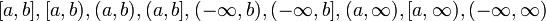# Difference between revisions of "Continuous functions form a vector space"

Suppose$I$ is an interval (possibly open, closed, or infinite from the left side and possibly open, closed, or infinite from the right side -- so it could be of the form$[a,b],[a,b),(a,b),(a,b],(-\infty,b),(-\infty,b],(a,\infty),[a,\infty),(-\infty,\infty)$). A continuous function on$I$ is a function on$I$ that is continuous at all points on the interior of$I$ and has the appropriate one-sided continuity at the boundary points (if they exist).
The continuous functions on$I$ form a real vector space, in the sense that the following hold:
• Additive: A sum of continuous functions is continuous: If$f,g$ are both continuous functions on$I$, so is$f + g$.
• Scalar multiplies: If$\lambda \in \R$ and$f$ is a continuous function on$I$, then$\lambda f$ is also a continuous function on$I$.